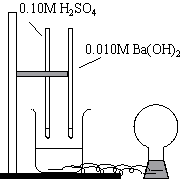## 3.5 Electrical Conductivity as an Endpoint Indicator

Chemical Concepts Demonstrated: Titration, electrolytes

Demonstration:

 .010M H2SO4 is added to the beaker of water. Note the conductivity of the solution. Add .010M Ba(OH)2 to the solution, and use the light as the indicator of the equivalence point of the titration. Ba(OH)2 is added dropwise until the indicator changes color as well; then add excess.Observations:

The light bulb glows when H2SO4 is initially added.  The light bulb dims and eventually goes out as Ba(OH)2 is added.  The indicator, however, doesn't change until excess Ba(OH)2 is added, at which point the light begins to glow again.

Explanations (including important chemical equations):

The reaction is as follows:

H2SO4 (aq) + Ba(OH)2 (aq) -> BaSO4 (s) + 2 H2O (l)

The light bulb glows when either H2SO4 or BaSO4 is in excess (i.e. when an ionic solution is present).  The light bulb glows when the H2SO4 is initially added, but slowly dims as the BaSO4 is added.  When the light goes out, and the solution is no longer ionic in any way, the equivalence point is reached.

It is important to note that this is not the point at which the indicator changes color.  The color changes at the endpoint, which occurs after the equivalence point.  The indicator changes color when excess BaSO4 is added.  The light also comes back on at this point (an ionic solution is present again).